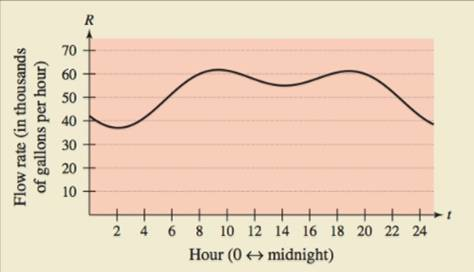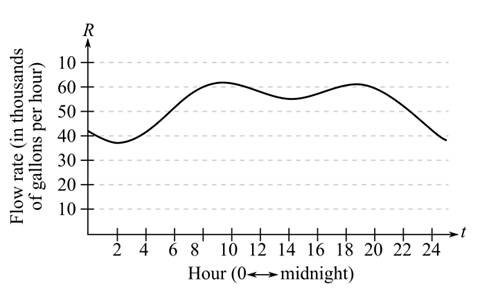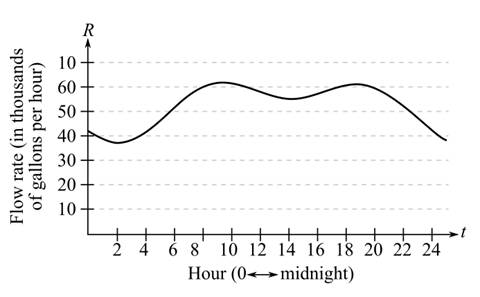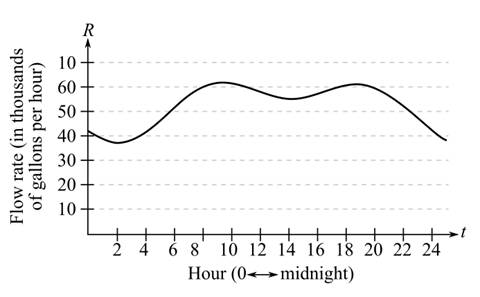Chapter 4.5, Problem 84E

Chapter
Section
Textbook Problem

HOW DO YOU SEE IT? The graph shows the flow rate of water at a pumping station for one day.(a) Approximate the maximum flow rate at the pumping station. At what time does this occur?(b) Explain how you can find the amount of water used during the day.(c) Approximate the two-hour period when the least amount of water is used. Explain your reasoning.

(a)

To determine

To calculate: The maximum flow rate and the time of its occurrencefrom the provided graphExplanation

Given:

The provided graph is:

Calculation:

The maximum flow will be the point on which the graph is at its maximum height...

(b)

To determine
The explanation to calculate the amount of water used in the day from the provided graph(c)

To determine

To calculate: The two-hour time period when the least amount of water is used and also explain the reason from the provided graphStill sussing out bartleby?

Check out a sample textbook solution.

See a sample solution

The Solution to Your Study Problems

Bartleby provides explanations to thousands of textbook problems written by our experts, many with advanced degrees!

Get Started

Sketch the graphs of the equations in Exercises 512. yx=1

Finite Mathematics and Applied Calculus (MindTap Course List)

Differentiate the function. f(x)=ln1x

Single Variable Calculus: Early Transcendentals, Volume I

Sketch the graph of the function. 50. g(x) = ||x| 1|

Single Variable Calculus: Early Transcendentals

True or False: f(x) = 3x x3 is concave down for x 1.

Study Guide for Stewart's Single Variable Calculus: Early Transcendentals, 8th

Discovery and Writing If 53t=kt, find k.

College Algebra (MindTap Course List)